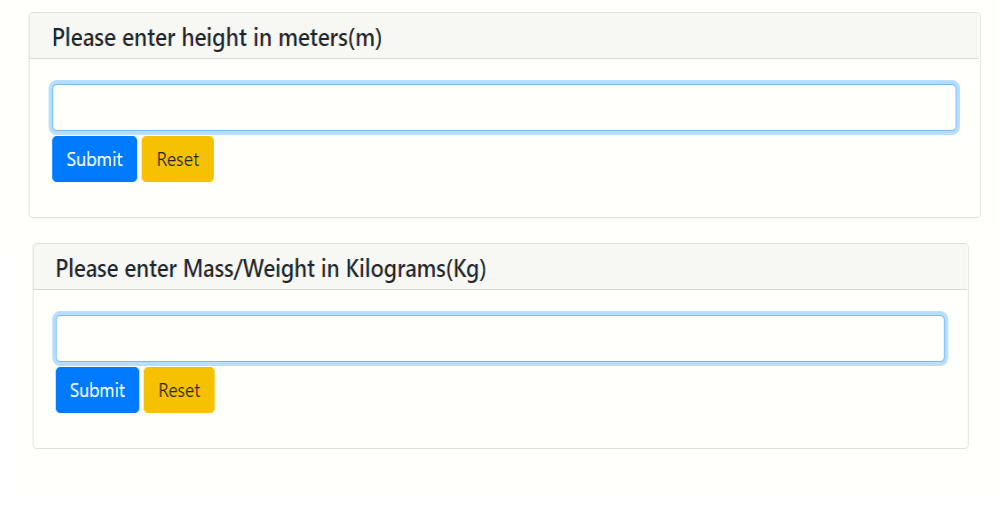# How to create BMI Calculator Web App using Python and PyWebIO ?

• Difficulty Level : Expert
• Last Updated : 19 Jul, 2022

In this article, we are going to create a BMI calculator using the PyWebIO module. This is a python module mostly used to create simple and interactive interfaces on the web using Python programming. It can be installed using the below command:

`pip install pywebio`

Before creating a calculator let’s take a brief understanding of BMI, The Body Mass Index (BMI) is the value obtained from dividing the weight of a person in Kg by its height square. The SI unit of BMI is kg/m2. Body Mass Index is directly proportional to the mass of an individual and inversely proportional to the square of the height of the individual.

Formula:

```BMI = Mass of person /  (height)2
where,
Mass in Kilograms(Kg), height in meters(m)```

Now, we create a Calculator of BMI using Python and a very interesting library PyWebIO.

### Stepwise Implementation:

Import the required modules. For using the python library PyWebIO we have to import some important modules from this library:

## Python

 `# importing modules` `from` `pywebio.``input` `import` `*` `from` `pywebio.output ``import` `*`

As we can see in the above python program that we first import the required modules from the PyWebIO library. Then we create a class calculation in which we create BMIcalculator() method classifies a person based on the BMI passed as parameter

## Python

 `# classify and compute BMI` `class` `calculation:`   `    ``# defining method` `    ``def` `BMIcalculator(``self``, Height, Mass):` `        `  `        ``# compute BMI` `        ``BMI ``=` `(Mass)``/``(Height``*``Height)` `        `  `        ``# classify` `        ``for` `t1, t2 ``in` `[(``16``, ``'severely underweight'``), ` `                       ``(``18.5``, ``'underweight'``), ` `                       ``(``25``, ``'normal'``), (``30``, ``'overweight'``), ` `                       ``(``35``, ``'moderately obese'``), ` `                       ``(``float``(``'inf'``), ``'severely obese'``)]:` `          `  `            ``if` `BMI <``=` `t1:` `                ``put_text(``'Your BMI is'``, BMI, ``'and the person is :'``, t2)` `                ``break`

After that from the user we take two inputs as for calculation we need height, mass, and then we calculate the result BMI by using the BMI formula and pass them as parameters in BMIcalculator() which computes BMI and classifies the weight category as per as result of BMI.

## Python

 `# height input` `Height ``=` `input``(``"Please enter height in meters(m)"``, ``type``=``FLOAT``)`   `# Mass input` `Mass ``=` `input``(``"Please enter Mass/Weight in Kilograms(Kg)"``, ``type``=``FLOAT``)`   `obj ``=` `calculation()` `obj.BMIcalculator(Height, Mass)`

Below is the complete program:

## Python

 `# importing modules` `from` `pywebio.``input` `import` `*` `from` `pywebio.output ``import` `*`   `# classify person` `class` `calculation:`   `    ``# defining method` `    ``def` `BMIcalculator(Height, Mass):`   `        ``for` `t1, t2 ``in` `[(``16``, ``'severely underweight'``),` `                       ``(``18.5``, ``'underweight'``),` `                       ``(``25``, ``'normal'``),` `                       ``(``30``, ``'overweight'``),` `                       ``(``35``, ``'moderately obese'``),` `                       ``(``float``(``'inf'``), ``'severely obese'``)]:` `            ``if` `BMI <``=` `t1:` `                ``put_text(``'Your BMI is'``, BMI, ``'and the person is :'``, t2)` `                ``break`   `# classify and compute BMI` `class` `calculation:`   `    ``# defining method` `    ``def` `BMIcalculator(``self``, Height, Mass):`   `        ``# compute BMI` `        ``BMI ``=` `(Mass)``/``(Height``*``Height)`   `        ``# classify` `        ``for` `t1, t2 ``in` `[(``16``, ``'severely underweight'``),` `                       ``(``18.5``, ``'underweight'``),` `                       ``(``25``, ``'normal'``), (``30``, ``'overweight'``),` `                       ``(``35``, ``'moderately obese'``),` `                       ``(``float``(``'inf'``), ``'severely obese'``)]:`   `            ``if` `BMI <``=` `t1:` `                ``put_text(``'Your BMI is'``, BMI, ``'and the person is :'``, t2)` `                ``break`     `# height input` `Height ``=` `input``(``"Please enter height in meters(m)"``, ``type``=``FLOAT``)`   `# Mass input` `Mass ``=` `input``(``"Please enter Mass/Weight in Kilograms(Kg)"``, ``type``=``FLOAT``)`   `obj ``=` `calculation()` `obj.BMIcalculator(Height, Mass)`

Output:As in the output we take Height (1.7 m) and Mass (60 Kg) at the time of input, and we can see in the output the calculated BMI and their type.

My Personal Notes arrow_drop_up
Recommended Articles
Page :